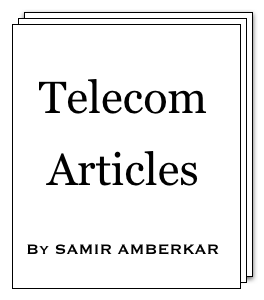online LTE test online C test Updated or New CDF explained5G Page5G Intent (a presentation)5G Intent (article)CV2X PageA Look at CV2X (a presentation)

 About Feedback Information Theory Modulation Multiple Access OSI Model Data Link layer SS7 Word about ATM GSM GPRS UMTS WiMAX LTE 5G Standard Reference Reference books Resources on Web Miscellaneous CV2X Mind Map Perl resources Magic MSC tool Bar graph tool C programming ASCII table Project Management Simple Google box HTML characters Site view counter 7 5 0 , 5 4 0 (May-2010 till Mar-2019)Solving modulation equations

Solving modulation equations [Under GSM > GMSK modulation]

In this article, we will solve the modulation equations from earlier article so that we could plot it and get insight into mathematics behind.

Let us start with Convolution of Gaussian function h(t) with rectangular pulse of width T.

 g(t) = h(t) * rect( t ) T

g(t) can be written as:

 . t+T/2 . g(t) = ∫ h(u) du . t-T/2 .

and further be evaluated as

 g(t) = 1 ( erf( t+T/2 ) - erf( t-T/2 ) ) 2T δT√2 δT√2

Error function (erf) is defined as below:

. . x . . .
erf(x) =

 2 √π

e

-t2

dt

. . -∞ . . .
Integral of erf is:

erf(ax+b) dx =

 1 a

(ax+b) erf(ax+b) +

 1 a.√π

e

-(ax+b)2

gnuplot has function erf, so it is possible to plot equations involving erf.Next step is Integral:

 . t' . G(t') = πh . ∫ g(u) du . -∞ .

Using above formula, we can evaluate it as below:

G(t') = πh . (

 t+T/2 2T

erf(

 t+T/2 δT.√2

) +

 δ √2π

e

-(

 t+T/2 δT.√2

)2

-

 t-T/2 2T

erf(

 t-T/2 δT.√2

) -

 δ √2π

e

-(

 t-T/2 δT.√2

)2

)

Now φ(t') and x(t') can be plot (with gnuplot).

In next article, we will take a small example of 1 bit change.

References: GSM book by Mouly and Pautet, Wolfram online integrator, and Handbook of mathematical functions by Abramowitz and Stegun